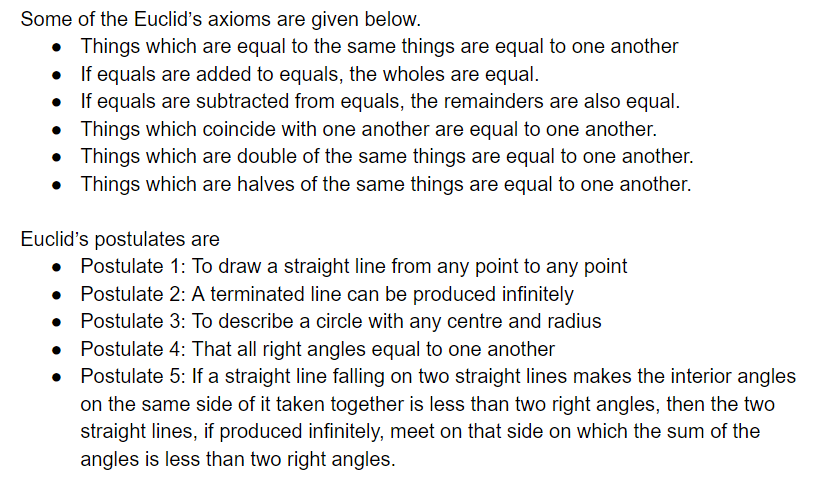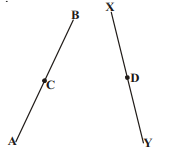# AP Board Class 9 Maths Chapter 3 The Elements of Geometry

Andhra Pradesh board Class 9 Maths Chapter 3, helps students to understand the History and the roots of Geometry. Students will learn about the different schools of thought that have contributed to the development of Geometry and basically compare it with modern Geometry. Notably, some of the important topics that are covered in this chapter are;

• Euclid’s Element
• Non-euclidean Geometry
• Some Axioms and Postulates

## Geometry History

The word geometry is taken from the Greek word ‘geo’ meaning earth and ‘metrein’, which translates to the term measure. The existence of geometry can be traced back to 2nd millennium BC and was first developed in the form of basic principles regarding areas, volumes, lengths, and angles, which were all used to meet some practical need in astronomy, surveying, construction, and various other crafts. Some of the important names and topics discussed include Bakshali manuscript, Indus Valley civilization ‘Sulba Sutras, Pythagoras of Ionia, Euclid of Alexandria.

### Euclid’s Elements

Euclid’s Elements is one of the most popular Mathematical work recorded in History and it even enjoys the status of being the world’s oldest and continuously used mathematical textbook. The notions of point, curves, line, plane, surface were derived from this thought.

### Some Axioms and Postulates

Axioms and postulates are the foundation stones on which the structure of geometry has been developed.Students can have a quick look at some of the chapter questions along with their solutions below and get a better understanding of the topic;

Question 1: How many dimensions a solid have?

Solutions: A solid has three dimensions, namely length, height and breadth.

Question 2: In the given figure, we have AC = XD, C and D are midpoints of AB and XY respectively. Prove that AB = XYSolution: Given AB = 2AC and XY = 2XD (taking c and d midpoints)

and AC = XD (given)

Therefore, AB = XY

Question 3: How many books are there in Euclid’s Elements?

Solution: There are 13 books.

Question 4: What is a straight line?

Solution: A straight line is a line which lies evenly with the points on itself.

Question 5: _________ is a surface which lies evenly with the straight lines on itself

Solution: A plane surface

Stay tuned to BYJU’s to know more about AP SSC Board and access various study materials, including syllabus, exam timetable and more.